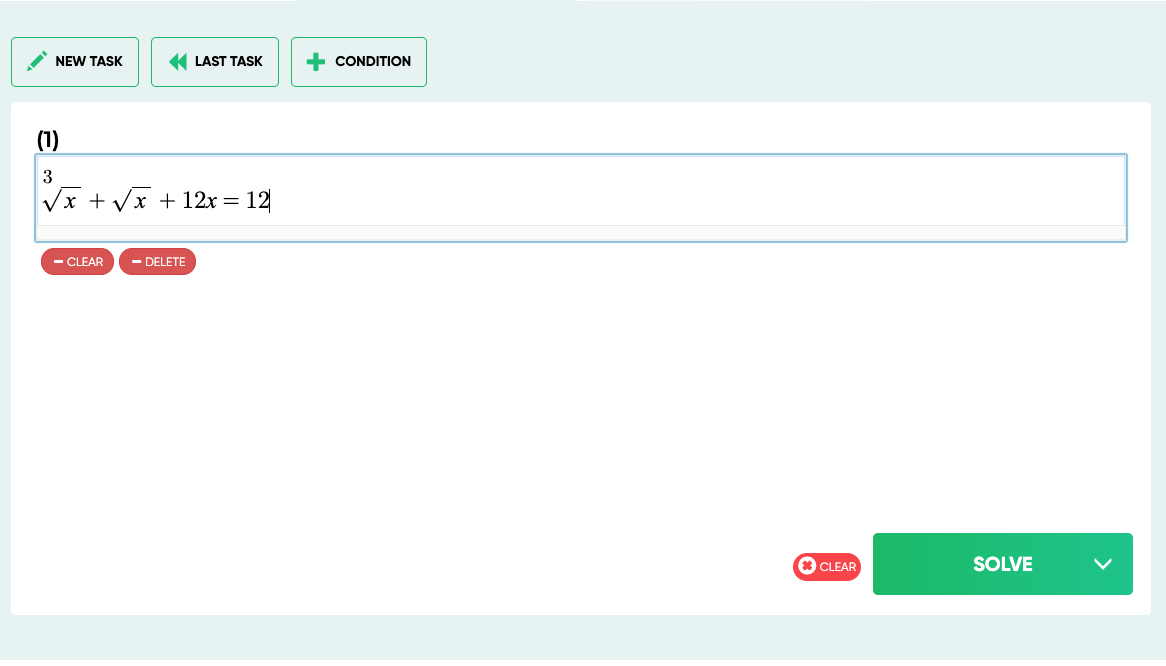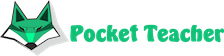# The history of the emergence and development of geometry

Geometry is one of the most ancient branches of mathematics. It is the study of points, lines, planes, closed planes and solid bodies. With knowledge of geometry, you can draw and describe almost any subject. Well, if you want to master the skill of calculating algebra , our resource will help you with this.## Stages of science formation

It is believed that people first started using geometry when the Egyptian pharaoh decided to tax farmers growing crops on the banks of the Nile. In order to determine the amount, it was necessary measure the area of ​​each site.

Around 2900 BC. e. the first pyramid was built in Egypt, for the construction of which people also knowledge of geometry was required. The most ancient formula for determining the area of ​​a triangle was made in 2000 BC. B.C.

The basic principles of geometry were developed by the Greeks around 600 - 400 BC. BC when Thales of Miletus proved the proportionality of the sides of such triangles. Quite a large contribution to the development of geometry made by Pythagoras. It was he who became the author of the world famous theorem, which proves that the squares the legs of a right-angled triangle add up to the square of the hypotenuse.

The ancestor of modern geometry is considered Euclid of Alexandria, who introduced the mathematical rigor and method of axioms, allowing solving math problems of various kinds. After that, into science no more significant updates were made until Rene Descartes, who became the creator of so called analytic geometry. This science studies the location of a geometric figure in a coordinate system for illustrating proofs and obtaining data using the principles of algebra.

Karl Friedrich Gauss made an important contribution to the formation of non-Euclidean geometry. Its main postulate states that through a certain point on a straight line there is one single line that is parallel this line. With the help of his notes, Einstein later developed his theory of relativity.Our artificial intelligence solves complex math problems in seconds.

We will solve your exam, homework, olympiad problems with detailed steps. You will need just to copy the solution.